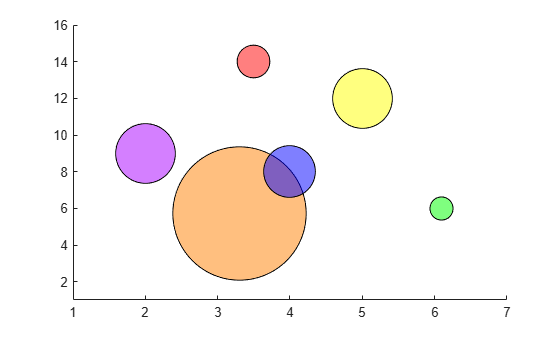# prism

Prism colormap array

## Syntax

``c = prism``
``c = prism(m)``

## Description

````c = prism` returns the prism colormap as a three-column array with the same number of rows as the colormap for the current figure. If no figure exists, then the number of rows is equal to the default length of 256. Each row in the array contains the red, green, and blue intensities for a specific color. The intensities are in the range [0,1], and the color scheme is a repeated sequence of the rainbow colors.```

example

````c = prism(m)` returns the colormap with `m` colors.```

## Examples

collapse all

Create a scatter plot with the default colors.

```x = [3.5 3.3 5 6.1 4 2]; y = [14 5.7 12 6 8 9]; sz = 100*[6 100 20 3 15 20]; c = [1 2 3 4 5 6]; scatter(x,y,sz,c,'filled','MarkerEdgeColor','k','MarkerFaceAlpha',.5); xlim([1 7]); ylim([1 16]);```Get the prism colormap array with six entries. Then replace the colormap in the scatter plot.

```c = prism(6); colormap(c);```## Input Arguments

collapse all

Number of colors, specified as a scalar integer value. The default value of `m` is equal to the length of the colormap for the current figure. If no figure exists, the default value is 256.

Data Types: `single` | `double`

## Version History

Introduced before R2006a

expand all

Behavior changed in R2019b